In this tutorial we demonstrate another adaptive solution of free surface problems on unstructured meshes, using the example of a droplet propagating along a straight channel. The problem is extremely similar to the propagation of a bubble in a channel tutorial . Thus, we shall only discuss the differences from that tutorial. The key physical difference is that instead of the uniform pressure state in an inviscid bubble, the droplet consists of a viscous fluid that can support internal stress variations.

# The example problem

We illustrate the solution of the unsteady 2D Navier-Stokes equations by considering the propagation of a single droplet along a straight channel as shown in the sketch below. The non-dimensionalisation is the same as in the bubble tutorial, and we choose the viscosity and density of the surrounding liquid to be the reference values.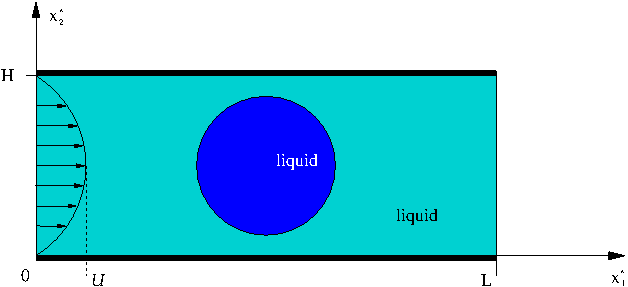The problem setup.

The governing equations and boundary conditions are the same as those in the
bubble tutorial. The only difference is that the Navier–Stokes equations must also be solved within the droplet. In fact, this is a two-fluid problem, a class of problems that is first introduced in another tutorial.

The constraint that the droplet volume remains constant must be enforced explicitly in the static case, as in the bubble problem. In the time-simulations, however, a constant drop volume is implicitly enforced by the continuity equation. For the bubble, the continuity equation is not solved within the interior, which is why the volume constraint must always be explicitly enforced in that case.

# Implementation

We use the same method as in the bubble problem, an ALE-based finite-element method with a pseudo-elastic node-update procedure. In this case, there is a pressure jump across the interface between the fluids, which means that we use triangular Crouzeix–Raviart elements rather than the continuous-pressure Taylor–Hood elements. Again, we impose the kinematic and dynamic boundary conditions with `FaceElements`. The volume constraint for the static problem is also imposed in a similar way: we attach `LineVolumeConstraintBoundingSolidElements` to the droplet surface and create an additional `VolumeConstraintElement`. Here, a pressure degree of freedom within the droplet is hijacked, see another tutorial, to be used as the unknown associated with the volume constraint. Once the static initial problem has been solved, the "volume constraint" elements are deleted and the pressure degree of freedom is unhijacked.

# Results

We perform the simulation in a two-stage procedure. We start by performing a steady solve with the inflow switched off. This deforms the droplet into its steady state (approximately) circular configuration with the required volume. The actual time-dependent simulation is then performed with an impulsive start from this configuration.

The figure below shows the location of the droplet and mesh (upper figure) and a contour plot of the pressure distribution with overlaid velocity vectors of the difference between the background Poiseuille flow and the velocity field (lower figure). The figure is a snapshot for the parameters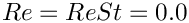,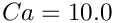and a droplet that is ten times as viscous as the surrounding liquid.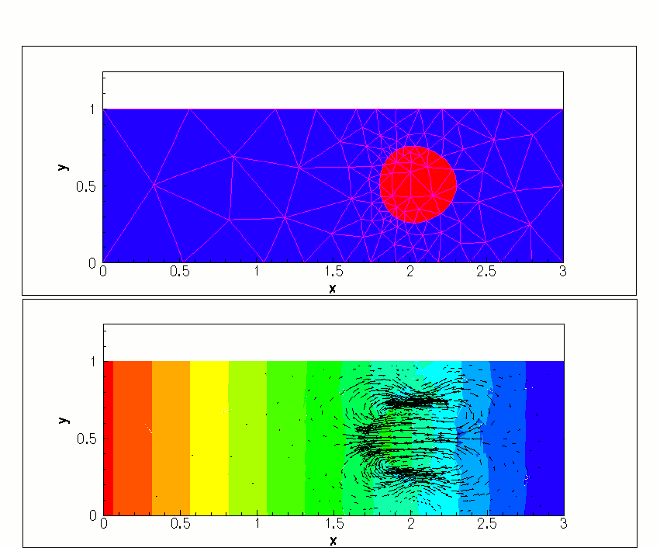Snapshot of the flow field (velocity vectors and pressure contours) for a propagating droplet.

# Global parameters

The namespace containing the dimensionless parameters contains an additional viscosity ratio parameter, compared to that in the bubble problem .

/// Viscosity ratio of the droplet to the surrounding fluid
double Viscosity_ratio = 10.0;
double Viscosity_ratio
Viscosity ratio of the droplet to the surrounding fluid.

# The driver code

The first difference from the bubble problem is that the steady solver does not converge when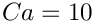. Instead, we start with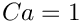, solve the steady problem, setand then resolve.

//Set the Capillary number to 10 and resolve
double Ca
Capillary number.

After documenting the solution, we remove the (explicit) volume constraint and then the remainder of the code is identical to that in the bubble tutorial.

// Switch off volume constraint
problem.remove_volume_constraint();

# The problem class

Other than trivial name changes from "bubble" to "drop", there are a few significant changes between this problem and that in the
bubble tutorial. There is an additional boolean `Use_volume_constraint` and an additional function `remove_volume_constraint()`, which are used to manage the switch from the explicit enforcement of the volume constraint in the static case to the implicit enforcement in the time simulations. The other key difference is that we use `Triangle's` region attributes to distinguish the elements inside the droplet from those outside. The default behaviour is that all elements are in region 0, but we label those element within the drop as region 1.

# The problem constructor

The construction of the mesh proceeds in exactly the same way as in bubble tutorial, except that we add a region tag "1" to label the elements within the droplet (so we specify a coordinate within the drop) and we must tell `Triangle` to use the assigned attributes.

// Define the region

The remainder of the constructor is the same as the other tutorial.

# Problem setup

When the bulk elements are made fully functional, we add the pointer to the viscosity ratio to all elements in the drop (region 1).

//For the elements within the droplet (region 1),
//set the viscosity ratio
n_element = Fluid_mesh_pt->nregion_element(1);
for(unsigned e=0;e<n_element;e++)
{
// Upcast from GeneralisedElement to the present element
ELEMENT* el_pt =
dynamic_cast<ELEMENT*>(Fluid_mesh_pt->region_element_pt(1,e));
el_pt->viscosity_ratio_pt() = &Problem_Parameter::Viscosity_ratio;
}

# Generation of face elements

As usual we impose the kinematic and dynamic boundary condition at the interface by attaching `FaceElements` to the relevant boundaries of the bulk elements. However, we must be careful to add only a single layer of elements. If we use the standard "boundary element" functions then we will be creating face elements on both sides of the internal boundary. Instead, we use the elements adjacent to the boundary within region 0, which ensures that only a single layer of interface elements are added.

//============start_of_create_free_surface_elements===============
/// Create elements that impose the kinematic and dynamic bcs
/// for the pseudo-solid fluid mesh
//=======================================================================
template<class ELEMENT>
{
//Loop over the free surface boundaries
unsigned nb=Fluid_mesh_pt->nboundary();
for(unsigned b=First_drop_boundary_id;b<nb;b++)
{
// Note: region is important
// How many bulk fluid elements are adjacent to boundary b in region 0?
unsigned n_element = Fluid_mesh_pt->nboundary_element_in_region(b,0);
// Loop over the bulk fluid elements adjacent to boundary b?
for(unsigned e=0;e<n_element;e++)
{
// Get pointer to the bulk fluid element that is
// adjacent to boundary b in region 0
ELEMENT* bulk_elem_pt = dynamic_cast<ELEMENT*>(
Fluid_mesh_pt->boundary_element_in_region_pt(b,0,e));
//Find the index of the face of element e along boundary b in region 0
int face_index = Fluid_mesh_pt->face_index_at_boundary_in_region(b,0,e);
// Create new element
ElasticLineFluidInterfaceElement<ELEMENT>* el_pt =
new ElasticLineFluidInterfaceElement<ELEMENT>(
bulk_elem_pt,face_index);
// Add it to the mesh
el_pt->set_boundary_number_in_bulk_mesh(b);
//Specify the capillary number
el_pt->ca_pt() = &Problem_Parameter::Ca;
}
}
}
// end of create_free_surface_elements
void create_free_surface_elements()
Create free surface elements.

The volume constraint elements are only created if the boolean flag `Use_volume_constraint` is true (the default on construction of the problem). We hijack the pressure degree of freedom associated with the first element in region 1 and then the construction of the elements again uses the regions to ensure that a single layer of elements is created.

//============start_of_create_volume_constraint_elements=================
/// Create elements that impose volume constraint on the drop
//=======================================================================
template<class ELEMENT>
{
// Do we need it?
if (!Use_volume_constraint) return;
// Store pointer to element whose pressure we're trading/hi-jacking:
// Element 0 in region 1
Hijacked_element_pt= dynamic_cast<ELEMENT*>(
Fluid_mesh_pt->region_element_pt(1,0));
// Set the global pressure data by hijacking one of the pressure values
// from inside the droplet
Drop_pressure_data_pt=Hijacked_element_pt->
// Build volume constraint element -- pass traded pressure to it
Vol_constraint_el_pt=
new VolumeConstraintElement(&Problem_Parameter::Volume,
Drop_pressure_data_pt,
//Provide a reasonable initial guess for drop pressure (hydrostatics):
Initial_value_for_drop_pressure);
// Add volume constraint element to the mesh
//Loop over the free surface boundaries
unsigned nb=Fluid_mesh_pt->nboundary();
for(unsigned b=First_drop_boundary_id;b<nb;b++)
{
// Note region is important
// How many bulk fluid elements are adjacent to boundary b in region 0?
unsigned n_element = Fluid_mesh_pt->nboundary_element_in_region(b,0);
// Loop over the bulk fluid elements adjacent to boundary b?
for(unsigned e=0;e<n_element;e++)
{
// Get pointer to the bulk fluid element that is
// adjacent to boundary b in region 0?
ELEMENT* bulk_elem_pt = dynamic_cast<ELEMENT*>(
Fluid_mesh_pt->boundary_element_in_region_pt(b,0,e));
//Find the index of the face of element e along boundary b in region 0
int face_index = Fluid_mesh_pt->face_index_at_boundary_in_region(b,0,e);
// Create new element
ElasticLineVolumeConstraintBoundingElement<ELEMENT>* el_pt =
new ElasticLineVolumeConstraintBoundingElement<ELEMENT>(
bulk_elem_pt,face_index);
//Set the "master" volume constraint element
el_pt->set_volume_constraint_element(Vol_constraint_el_pt);
// Add it to the mesh
}
}
}
// end of create_volume_constraint_elements
void create_volume_constraint_elements()
Create elements that impose volume constraint on the drop.
double Volume
Volume of the bubble (negative because it's outside the fluid!)

# Removal of the volume constraint

The function `remove_volume_constraint()`, resets the boolean flag to false, clears the hijacked data, deletes the volume constraint elements and mesh and then removes the volume constraint mesh from the problem's list of sub meshes, before reassigning the equation numbers.

/// Change the boundary conditions to remove the volume constraint
void remove_volume_constraint()
{
// Ignore all volume constraint stuff from here onwards
Use_volume_constraint=false;
//Unhijack the data in the internal element
Hijacked_element_pt->unhijack_all_data();
//Delete the volume constraint elements
delete_volume_constraint_elements();
// Internal pressure is gone -- null it out
Drop_pressure_data_pt=0;
// Kill the mesh too
delete Volume_constraint_mesh_pt;
Volume_constraint_mesh_pt=0;
//Remove the sub meshes
this->flush_sub_meshes();
//Rebuild the global mesh
this->rebuild_global_mesh();
//Renumber the equations
std::cout << "Removed volume constraint to obtain "
<< this->assign_eqn_numbers() << " new equation numbers\n";
}

The computation of the initial static solution means that we must still include the calls to the `create_volume_constraint_elements()` and `delete_volume_constraint_elements()` in `actions_before_adapt()` and `actions_after_adapt()`. We have chosen to have the functions return immediately if the volume constraint is not being enforced, rather than using `if` blocks within the `actions_before/after_adapt`() functions.

## Exercises

1. Explore what happens as the viscosity ratio is varied. Are the results as you expect? Does the solution tend to a steadily propagating state? Does the solution approach the bubble solution as this viscosity ratio tends to zero? (Is this a sensible limit to take?)

2. How could you modify the code to compute the steadily-propagating solutions directly, rather than using time simulation?